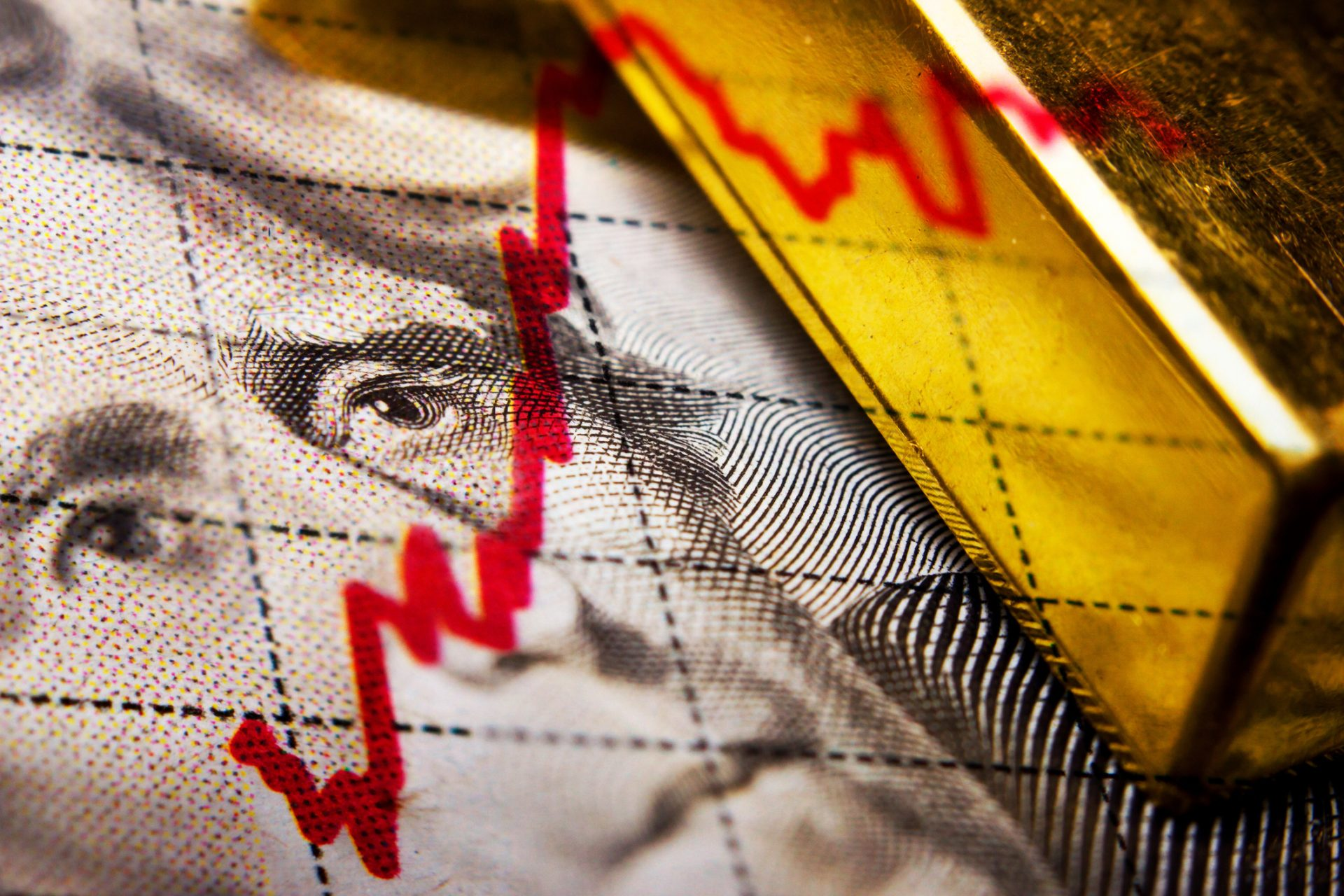# The Relationship Between Bond Prices and Interest Ratesclaffra / Getty Images/iStockphoto

Understanding the inverse relationship between bond prices and interest rates can be a little confusing for new investors. However, taking an in-depth look at the various characteristics of bonds, bond prices and interest rates can help any investor see the relationship clearer.

Bond prices and interest rates are closely related and can both be used to forecast economic activity, so investors should at least be aware of the basics: how interest rates affect bond prices, how to calculate bond prices and how yield-to-maturity rates come into play.

## Understanding Bond Prices and Interest Rates

While it may seem paradoxical, bond prices are inversely related to interest rates — bond prices will increase when interest rates fall and vice versa. Because of that inverse relationship, all bonds carry interest rate risk. While the risk is generally less than many other investments, understanding interest rate risk is crucial if you plan to buy bonds.

Interest rates on investments are often influenced by the federal funds rate, set by the Federal Open Market Committee. If the federal funds rate increases, interest rates on many other investments will also increase.

Building Wealth

Therefore, an increase in interest rates would cause new bonds to come onto the market with higher interest rates than bonds selling with lower interest rates. Since organizations still want to sell the bonds with lower interest rates, they will decrease the bond price to compete with the new bonds with higher interest rates.

This benefits the organization because their bonds likely continue to sell, and it helps investors because they’re not paying more than what the bond is worth relative to current interest rates.

## How To Calculate Bond Prices

There are a few factors to know when calculating bond prices, including:

• Coupon rate: The percentage of the par value redeemable at each period.
• Par value: The amount that is repaid at maturity. If a bond has a coupon rate, the investor will receive a coupon payment each period and the par value plus a coupon payment at maturity.
• YTM: The total interest rate a bond will have paid at maturity, including all interest or coupon payments and the par value.
• Periods to maturity: Equals how many coupon payments you will receive before maturity. They are typically paid annually but may also be semi-annually or quarterly.
Building Wealth

Many investors may use the following formula to calculate bond prices:

P(T0) = [PMT(T1) / (1+r)1] + [PMT(T2) / (1+r)2] + … + [(PMT(Tn) + FV) / (1+r)n]

• P(T0) = bond price at period zero
• PMT(Tn) = coupon payment at period n
• FV = par value
• r = yield to maturity
• n = number of periods

When considering bond prices, higher coupon rates, par values or periods to maturity will have higher prices. However, if a bond has a higher YTM, the bond price will be lower.

## Bond Prices vs. Yields

According to the Securities and Exchange Commission’s bulletin on interest rate risk, bond prices also have an inverse relationship with YTM rates. The yield will match the coupon rate when a bond is issued and sold at par value. However, if an investor pays less than the par value, their return would be more significant since the coupon payments and par value are fixed.

For example, consider a bond with a par value of \$1,000. If interest rates fall, an investor may need to pay \$1,100. Therefore at maturity, they will receive a smaller YTM. Similarly, if interest rates rise, the same bond may cost \$900. At maturity, the investor will receive a larger return.

Building Wealth

## Final Take

Since interest rates and bond prices have such a close relationship, those choosing to invest in bonds should keep up with market fluctuations to gauge the effects on investments.

However, interest rates and bond prices shouldn’t be the only considerations investors make when buying bonds. They must also consider additional factors, such as the risk profile of certain bonds, a bond’s prospectus, any costs or fees associated with buying the bond, the liquidity of each bond and how bonds fit into overall investing objectives.

Shrewd investors will do well to research thoroughly and learn about a bond’s coupon rates, maturity date and past performance, as it’s clear that rates, bond prices and yields can all be affected by several elements of investing.

Information is accurate as of July 18, 2022.

Building Wealth

Learn More